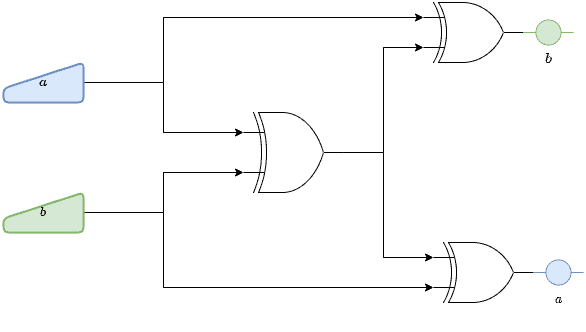## 1. Introduction

In this tutorial, we’ll study and understand the XOR swap of two variables.

## 2. XOR Operation

XOR is a bit operation. It accepts two operands and compares their values. XOR evaluates to 0 (or False) if the operands are the same. If they differ, it outputs outputs 1 (or True). Here’s its truth table:

``````

Usually, we denote XOR with.

### 2.1. Properties

XOR is commutative:It’s also associative:Its neutral element is zero:XORing anywith itself, we getas the result:So, each element is its own inverse with respect to XOR.

### 2.2. XOR and Numbers

We can define XOR for numbers by applying the bitwise XOR to the pairs of corresponding bits. Let’s takeandas an example. In the binary notation, we haveand. Now:We get that the result of the XOR operation onandis 29 (binary).

### 2.3. Why Do We Use XOR for Swapping?

In the old days of single-core CPU systems, memory access was a costly operation and CPU registers were very precious resources. So, we used XOR swapping to avoid costly memory dereferences and loading CPU register values with the stack as in the ordinary swap with a temporary variable.

## 3. XOR Swap Pseudocode

The most common use of XOR is to swap two variables without any auxiliary storage:

``

The following figure shows this operation as a circuit gate operation:As evident from the circuit diagram above, we feed inputsandto the first XOR gate in the middle. Then, we feed the output of the first XOR gate and inputto the second XOR gate on the top. This givesas the output. Lastly, we pass the output of the first XOR gate and inputto the third XOR gate (the one at the bottom). This gives usas the result. So, we find the values are swapped.

### 3.1. Explanation

Now, we are ready for a detailed explanation of three swapping steps from the pseudocode.

In the first instruction, we apply XOR onandand store the result back in. Let:Now, in the next step, we do the XOR onand the result of the first step (). Let the result be:Now, using the, associativity, inverse, and neutral-element properties, we get:Thus, at the end of step 2,is original value of. Since we assigned it to, we get thatis the original.

Going further, we move to step 3. Here, we apply XOR to the result of steps 1 and 2 (and):Now, using the properties of XOR, we get:Hence, at the end we have. So the swap went down successfully.

## 4. Problems

In the present days of massive pipelines and quad-core CPU systems, XOR swapping can slow down the whole system. Why? Because XOR swapping holds intermediate values and has the massive dependency of one step on another.

This is because the system can execute each step on different CPU cores. Thus, the system can store the result of each step in different CPU registers. However, this dependency on reading from a CPU register and writing to different CPU register could cause the whole pipeline to stall. As a result, a stalled pipeline puts the code on a slower path.

Almost all modern-day compilers perform swaps using temporary storage in a single instruction, so XOR swaps don’t benefit from compiler-time optimization.

## 5. Conclusion

In this article, we have gone through in detail how we swap two variables without using any auxiliary storage variable. We find XOR swap useful in the old days of single-core CPU systems but it slows down the execution in multicore CPU systems.